Foxtable(狐表)用户栏目专家坐堂 → TreeView1与表中不同步

共有1287人关注过本帖树形打印复制链接

# 主题：TreeView1与表中不同步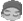cd_tdh
1楼 | 信息 | 搜索 | 邮箱 | 主页 | UC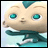加好友发短信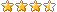TreeView1与表中不同步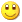Post By：2019/9/24 14:57:00 [只看该作者]

 老师，麻烦看看，窗口：导航维护，增加项、增加子项时，TreeView1的节点怎么让它和导航表同步。  以下内容是专门发给有点蓝浏览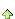2楼 | 信息 | 搜索 | 邮箱 | 主页 | UC加好友发短信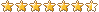Post By：2019/9/24 15:38:00 [只看该作者]

 增加项Dim dt As DataTable=DataTables(vars("editTable"))Dim r0 As Row =Tables(vars("editTable")).CurrentDim r As DataRow=dt.AddNewr("序号")=dt.sqlCompute("Max(序号)")+1'目录树Dim tr As WinForm.TreeView = e.Form.Controls("TreeView1")Dim sd As WinForm.TreeNode=tr.SelectedNode '当前选择的项Dim typ As String = "新分类" '分类Dim grp As String = "新分组" '分组Dim name As String = "新名称" '名称r("父键")=dt.sqlCompute("Max(父键)")+1r("分类")= typ & r("序号")Dim n = tr.Nodes.add(r("分类"))Dim s As String=r0("分类")tr.SelectedNode =tr.Nodes(s)sd.Expandr.Save增加子项Dim tr As WinForm.TreeView = e.Form.Controls("TreeView1")Dim sd As WinForm.TreeNode =tr.SelectedNode '当前选择的项If sd Is Nothing Then ReturnDim dt As DataTable=DataTables(vars("editTable"))Dim r0 As Row =Tables(vars("editTable")).CurrentDim typ As String ="新分类"  '分类Dim grp As String ="新分组" '分组Dim name As String ="新名称" '名称Select Case sd.level    Case 2        MessageBox.Show("这是最小节点,不能添加子项","提示",MessageBoxButtons.OK,MessageBoxIcon.Warning)        Return    Case 0        Dim r As DataRow=dt.AddNew        r("序号")=dt.sqlCompute("Max(序号)")+1        r("父键") = r0("父键")        r("分类")=  r0("分类")        r("分类图标") =  r0("分类图标")        r("分组")= grp & r("序号")        sd.nodes.Add(r("分类"),r("分组"),r("分类图标"))        Dim s As String=r("标题")        tr.SelectedNode =sd.Nodes(s)        sd.Expand        tr.Select        r.save    Case 1        Dim r1 As DataRow=dt.AddNew        r1("序号")=dt.sqlCompute("Max(序号)")+1        r1("父键") = r0("父键")        r1("分类")=  r0("分类")        r1("分类图标")=  r0("分类图标")        r1("分组")=  r0("分组")        r1("名称")=  name & r1("序号")        sd.nodes.add(r1("分类") & r1("分组"),r1("名称"),r1("分类图标"))        Dim s As String=r1("标题")        tr.SelectedNode =sd.Nodes(s)        sd.Expand        tr.Select        r1.saveEnd Selectcd_tdh
3楼 | 信息 | 搜索 | 邮箱 | 主页 | UC加好友发短信Post By：2019/9/24 16:05:00 [只看该作者]

 老师，增加项后，修改了分类名称，TreeView1增加的节点名称没有修改。增加子项后，新增加的子项也不是当前节点，与导航表中的行也没对应上。 增加项修改为这样： Dim dt As DataTable=DataTables(vars("editTable"))Dim r0 As Row =Tables(vars("editTable")).CurrentDim r As DataRow=dt.AddNewr("序号")=dt.sqlCompute("Max(序号)")+1'目录树Dim tr As WinForm.TreeView = e.Form.Controls("TreeView1")Dim sd As WinForm.TreeNode=tr.SelectedNode '当前选择的项Dim typ As String = "新分类" '分类r("父键")=dt.sqlCompute("Max(父键)")+1r("分类")= typ & r("序号")Dim n = tr.Nodes.add(r("分类"))Dim s As String=r("分类")tr.SelectedNode =tr.Nodes(s)sd.Expandr.Save 修改了分类名称，TreeView1增加的节点名称修改过来了，但是点击其他节点在点击这个新增加节点，节点与新增加的行又对应不上了，麻烦看看TreeView1中AfterSelectNode事件代码哪儿不对呢。 [此贴子已经被作者于2019/9/24 16:17:46编辑过]4楼 | 信息 | 搜索 | 邮箱 | 主页 | UC加好友发短信Post By：2019/9/24 16:26:00 [只看该作者]cd_tdh
5楼 | 信息 | 搜索 | 邮箱 | 主页 | UC加好友发短信Post By：2019/9/24 16:44:00 [只看该作者]

 老师，修改了还是与新增项一样，节点修改后，我点其他节点后再点刚修改的节点，还是没定位到导航表对应的行。 老师，这样修改对了：         Dim r As Integer        r = Tables(vars("editTable")).FindRow("[分类] Like '" & e.Node.text & "'") '从第一行开始查找        If r >= 0 Then '如果找到的话            Tables(vars("editTable")).Position = r '定位到找到的行.        End If [此贴子已经被作者于2019/9/24 17:18:56编辑过]cd_tdh
6楼 | 信息 | 搜索 | 邮箱 | 主页 | UC加好友发短信Post By：2019/9/25 14:05:00 [只看该作者]

 老师，麻烦看看上移按钮，我集合有点弄得混乱了，我上移项目管理后，导航表中没有把所有项目管理的父键变为 2 ，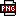此主题相关图片如下：qq截图20190925140257.png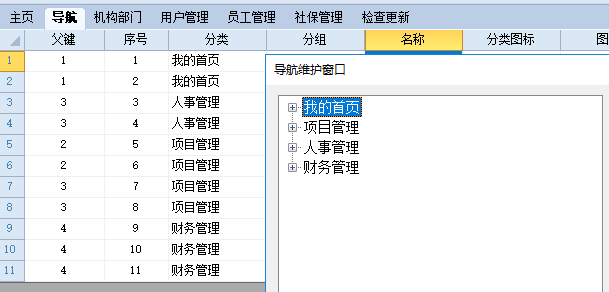代码如下： Dim dt As DataTable=DataTables(vars("editTable"))Dim tr As WinForm.TreeView = e.Form.Controls("TreeView1")Dim sd As WinForm.TreeNode=tr.SelectedNode '当前选择的项If sd Is Nothing Then ReturnDim i As Integer= sd.IndexSelect Case sd.level    Case 0        If i>0 Then            Dim r As  List(of  DataRow)= dt.Select("分类='" & sd.text & "'")            If r Is Nothing Then Return            For Each dr2  As DataRow In r                Dim sd1 As WinForm.TreeNode=tr.Nodes(i-1)                Dim r1 As List(of DataRow)= dt.Select("分类='" & sd1.text & "'")                For Each dr1  As DataRow In r1                    Dim s As Integer = dr1("父键")                    dr1("父键")=dr2("父键")                    dr2("父键")=s                Next            Next        End If        sd.MoveUp        tr.SelectEnd Select7楼 | 信息 | 搜索 | 邮箱 | 主页 | UC加好友发短信Post By：2019/9/25 14:16:00 [只看该作者]

 什么地方上移？按什么逻辑上移？cd_tdh
8楼 | 信息 | 搜索 | 邮箱 | 主页 | UC加好友发短信Post By：2019/9/25 14:25:00 [只看该作者]

 以下是引用有点蓝在2019/9/25 14:16:00的发言：什么地方上移？按什么逻辑上移？ 通过导航维护窗口，上移按钮，移动节点，导航表中对应分类父键相应改变。9楼 | 信息 | 搜索 | 邮箱 | 主页 | UC加好友发短信Post By：2019/9/25 14:28:00 [只看该作者]

 是同级别的节点上下交换顺序，还是把子节点移到父节点下面作为兄弟节点cd_tdh
10楼 | 信息 | 搜索 | 邮箱 | 主页 | UC加好友发短信Post By：2019/9/25 14:42:00 [只看该作者]

 以下是引用有点蓝在2019/9/25 14:28:00的发言：是同级别的节点上下交换顺序，还是把子节点移到父节点下面作为兄弟节点 同级别节点上下交换顺序总数 46 1 2 3 4 5 下一页